Next: Lagrange Techniques Up: Statistical Background Previous: Maximum Likelihood

## Least Squares Approaches

As we have mentioned previously, one way of de-correlating probabilities is to use a model. Take for example a set of data described by the function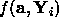where a defines the set of free parameters defining f andis the generating data set. If we now define the variation of the observed measurements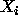about the generating function with some random error we can see that the probability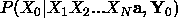will be equivalent to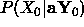as the model and generation point completely define all but the random error.

Choosing Gaussian random errors with a standard deviation of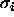gives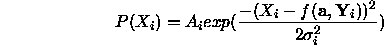where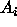is a normalisation constant. We can now construct the maximum likelihood function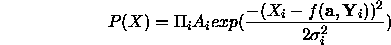which leads to the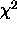definition of log likelihood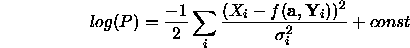This expression can be maximized as a function of the parameters a and this process is generally called a least squares fit. Whenever you encounter least squares there is therefore a built in assumption of independence and Gaussian distribution. In practical situations the validity of these assumptions should be checked by plotting the distribution of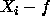to make sure that it is Gaussian.

The choice of a least squares error metric gives many advantages in terms of computational simplicity and later we will see that it is also used extensively for definitions of error covariance and optimal combination of data. However, the distribution of random variation on the observed data X is something that generally we have no initial control over and could well be arbitrary. This may initially be seen as an overwhelming problem but in most circumstances it is possible to make distributions handleable (Gaussian) by transformation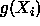and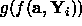, where g is chosen so that the initial distribution ofmaps to a Gaussian distribution in g .

One good example of this is in the location of a known object in 3D data derived from a stereo vision system. In the coordinate system where the viewing direction corresponds to the z axis, x and y measures have errors determined by image plane measurement. However, the depth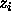for a given point is given by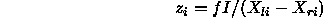where I is the interoccular separation, f is the focal length and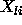and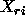are image plane measurements. Attempts to perform a least squares fit directly in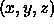space results in instability due to the non-Gaussian nature of thedistribution. However, transformation to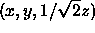yields Gaussian distributions and good results.

Under many circumstances, even after taking care to obtain Gaussian variation on the fitted quantities, there is still one final problem which needs to be addressed. This is the problem of fliers or outliers. Fliers are the name given to the data generated by any real system which do not conform to the assumed statistical distribution. These are generally caused by complete failure of the data measurement system and generated well away from the expected mean of the distribution. If ignored they can completely dominate the fitting process giving meaningless results. For example, measurement of the distance to an object pre-supposes that we have selected the correct object. The correct way to deal with these measures is to modify the expected probability distribution to include the long tails from fliers, this leads to the branch of numerical methods known as robust statistics. The simplest way to do this which allows us to continue to use standard methods for covariance estimation and optimal data combination, which assume Gaussian distribution, is to limit the contribution to thedistribution from any data point to some maximum value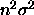. This makes the assumption that the statistical distribution is constant for any gearing point greater than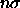from the expected position. Unfortunately this process precludes the use of standard least squares solution methods and solution must generally be iterative as the gearing point will vary for each data point during parameter estimation. This process is efficiently executed by the probabilistic Hough transform for small numbers of parameters .Next: Lagrange Techniques Up: Statistical Background Previous: Maximum Likelihood

Bob Fisher
Fri Mar 28 14:12:50 GMT 1997